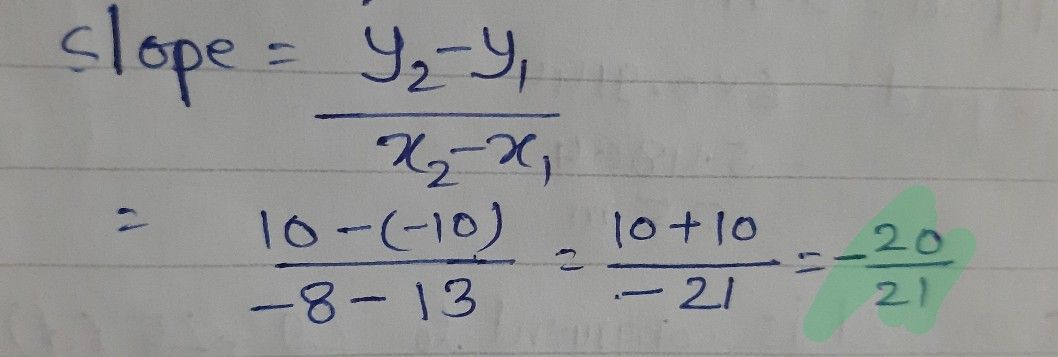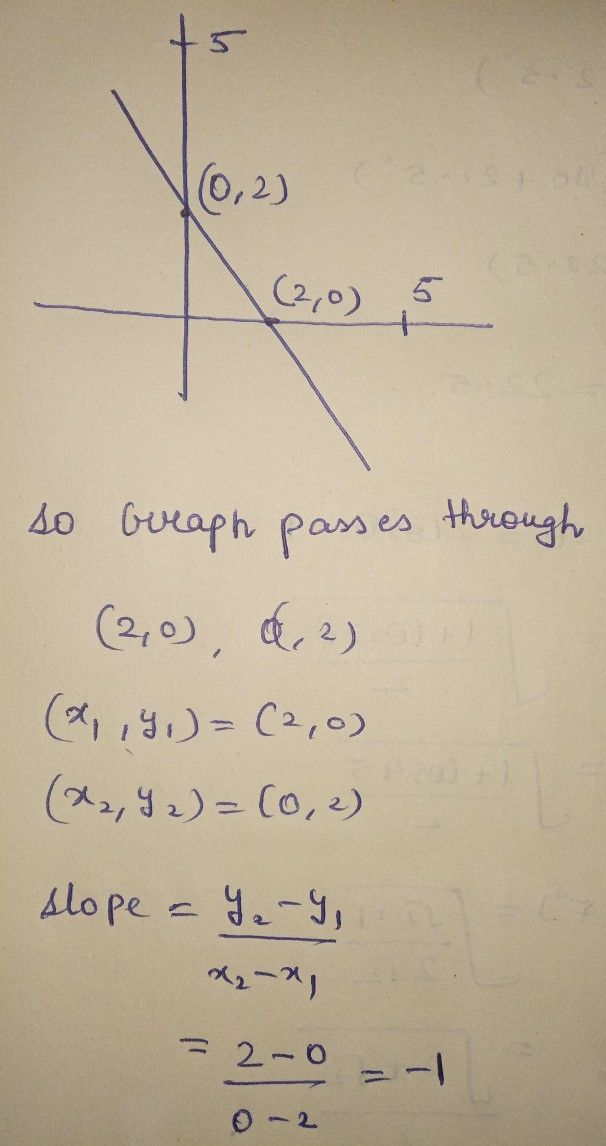Symbol
ProblemFind the $51010$ $1$ the line. $10$ $5$ $10$ 5. $-5$ $-10$ $-5$ $-10$
10th-13th grade
Algebra
SolutionQanda teacher - Aniruddh00Qanda teacher - GYANENDRAhave a great day thank you ? if you have any questions regarding this please ask